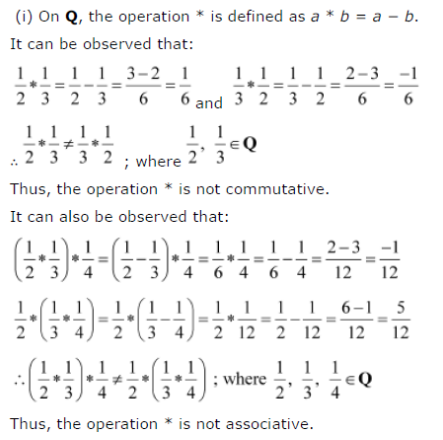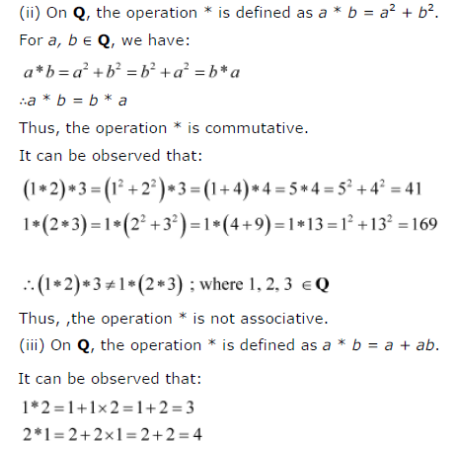# Let * be a binary operation on the set Q of rational numbers as follows:

Let * be a binary operation on the set Q of rational numbers as follows:
(i) a * b = a - b
(ii) a * b = a2 + b2
(iii) a * b = a + ab
(iv) a * b = (a - b )2
(v) a * b = ab /4
(vi) a * b = ab2
Find which of the binary operations are commutative and which are associative.